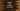# Find the Area of a circle in python : Python tutorial 28## Find the Area of a circle in python :

We know that the area of a circle is π r², where “r” is the radius of the circle. Since π is constant, we need only the radius value from the user to calculate the area. The value of π is 3.1415 up to fourth decimal places.

The radius is equal to half of the diameter. You can also modify this program to take the diameter as input from the user.

In this tutorial, we will learn how to find the area of a circle in python. Our program will take the radius as an input from the user, calculate the area and print it on the console.

The only thing we need apart from radius is π. We can store the π value in a separate constant variable or we can use the inbuilt ‘math’ module. I will show you both of these ways to calculate the area. Let’s take a look :

``````#method 1

PI = 3.14
r = float(input('Enter the radius of the circle :'))
area = PI * r * r

print("Area of the circle is : %.2f" %area)

#method 2

import math

r = float(input('Enter the radius of the circle :'))
area = math.pi * r * r

print("Area of the circle is : %.2f" %area)``````

You can also download these examples from here.

## Calculate circle area using constant π :

### Explanation :

1. In this example, we are storing the value of π in a constant variable PI. We are assigning its value ‘3.14’. You can change this value to any decimal point of π.
2. input() method is used to read the user input value. This method takes one string as its parameter. This string will be printed out to the user and the program will hold waiting for a user response. The return value of this method is of type string. We are converting this value to float by wrapping the result with float() method.
3. The value of the radius is stored in the variable ‘r’.
4. The area of the circle is calculated by using the constant PI and the radius ‘r’. The area is stored in the variable ‘area’.
5. We are printing the calculated area up to two decimal points by using the ‘%.2f’ operator.

## Using the math module :

In the example above, we have stored the value of π in a separate variable and calculated the area of the circle using this value. The main drawback of this method is that we need to create a separate variable to hold this value.

Python has one inbuilt module called ‘math’ that provides access to different mathematical functions and constants like trigonometric functions, logarithmic functions, hyperbolic functions etc. Constants like ‘pi’, ‘e’, ‘tau’, ‘inf’ and ‘nan’ are also defined inside this module.

The value of π is available in this module and we can use it by importing this module.

Let’s find the area of a circle using the math module of python :

### Output :

Here, we have imported the ‘math’ module and use the value of math.pi for π.

Similarly, we can also find out the circumference of a circle using `2πr`.

### Conclusion :

In this tutorial, we have learned how to find the area of a circle in python by taking the radius as an input. Try to run the examples shown above and drop one comment below if you have any queries.